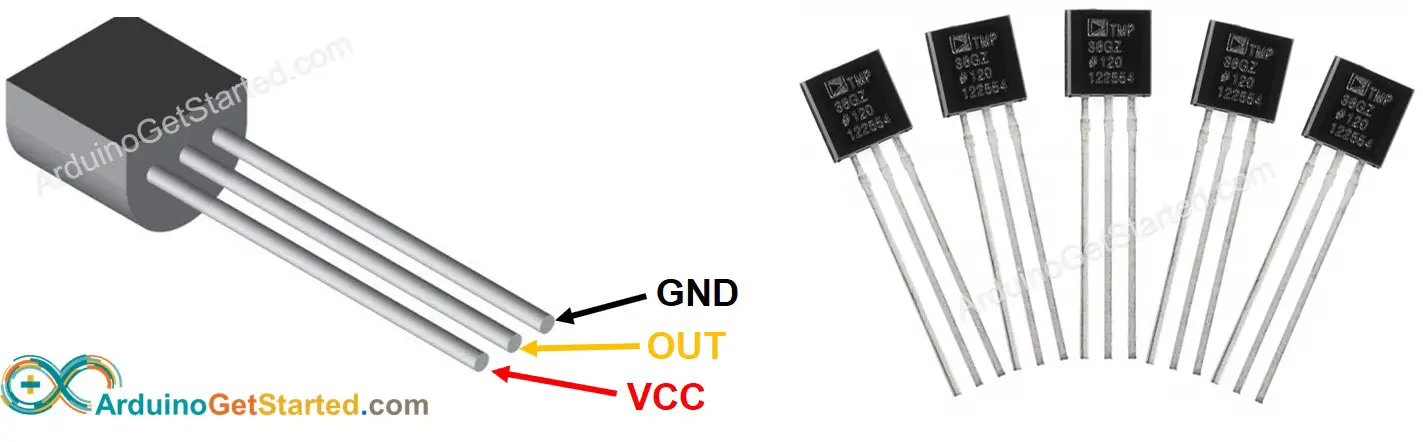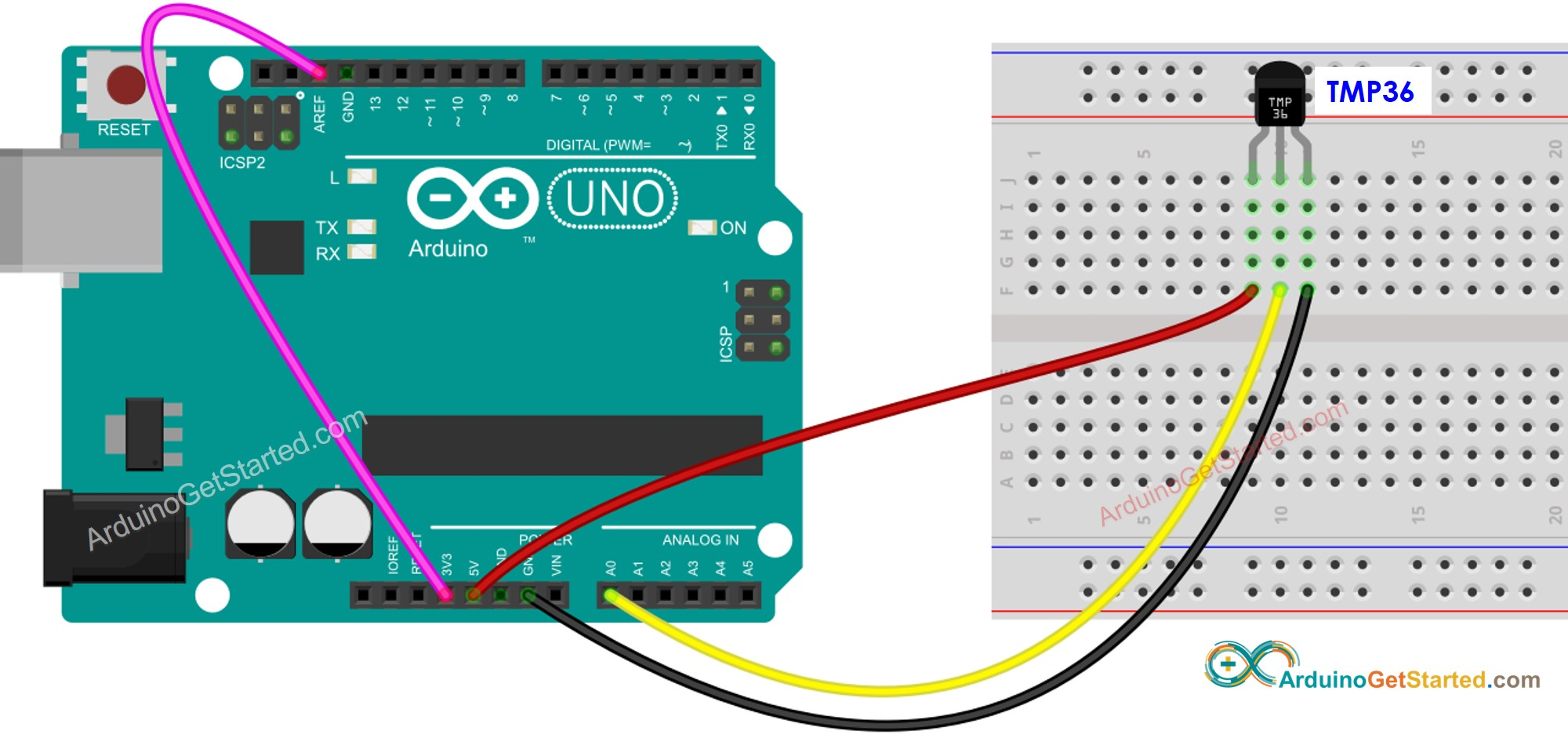# Arduino - TMP36 Temperature Sensor

## Hardware Required

Or you can buy the following sensor kit:

Please note: These are Amazon affiliate links. If you buy the components through these links, We will get a commission at no extra cost to you. We appreciate it.

### Pinout

TMP36 temperature sensor has three pins:

• GND pin: needs to be connected to GND (0V)
• VCC pin: needs to be connected to VCC (5V)
• +Vs pin: is the power supply for the sensor which can vary between 2.7V to 5.5V.
• Vout pin: signal pin gives the output voltage that is linearly proportional to the temperature, should be connected to a analog pin on Arduino.### How It Works

The TMP36 outputs the voltage linearly proportional to the Centigrade temperature. The output scale factor of the TMP36 is 10 mV/°C. It means that the temperature is calculated by dividing the voltage (mV) in output pin by 10.

## Wiring DiagramThis image is created using Fritzing. Click to enlarge image

## How To Program For TMP36 Temperature Sensor

• Get the ADC value from the temperature sensor by using analogRead() function.
• Convert the ADC value to voltage in voltage
// Replace 5.0 with 3.3, if you are using a 3.3V Arduino float voltage = adcVal * (5.0 / 1024.0);
• Convert the voltage to the temperature in Celsius
float tempC = (voltage - 0.5) * 100;
• (Optional) Convert the Celsius to Fahrenheit
float tempF = tempC * 9 / 5 + 32;

## Arduino Code

/* * Created by ArduinoGetStarted.com * * This example code is in the public domain * * Tutorial page: https://arduinogetstarted.com/tutorials/arduino-tmp36-temperature-sensor */ #define ADC_VREF 5.0 // in volt #define ADC_RESOLUTION 1024.0 #define PIN_TMP36 A0 void setup() { Serial.begin(9600); } void loop() { // get the ADC value from the TMP36 temperature sensor int adcVal = analogRead(PIN_TMP36); // convert the ADC value to voltage float voltage = adcVal * (ADC_VREF / ADC_RESOLUTION); // convert the voltage to the temperature in Celsius float tempC = (voltage - 0.5) * 100; // convert the Celsius to Fahrenheit float tempF = tempC * 9 / 5 + 32; // print the temperature in the Serial Monitor: Serial.print("TMP36 Temperature: "); Serial.print(tempC); // print the temperature in Celsius Serial.print("°C"); Serial.print(" ~ "); // separator between Celsius and Fahrenheit Serial.print(tempF); // print the temperature in Fahrenheit Serial.println("°F"); delay(1000); }

#### Quick Steps

• Copy the above code and open with Arduino IDE
• Click Upload button on Arduino IDE to upload code to Arduino
• Grasp the sensor by your hand
• See the result on Serial Monitor.
COM6
TMP36 Temperature: 26.31°C ~ 79.36°F TMP36 Temperature: 26.44°C ~ 79.59°F TMP36 Temperature: 26.50°C ~ 79.70°F TMP36 Temperature: 26.56°C ~ 79.81°F TMP36 Temperature: 27.06°C ~ 80.71°F TMP36 Temperature: 27.75°C ~ 81.95°F TMP36 Temperature: 28.37°C ~ 83.07°F TMP36 Temperature: 29.00°C ~ 84.20°F TMP36 Temperature: 29.56°C ~ 85.21°F TMP36 Temperature: 30.00°C ~ 86.00°F TMP36 Temperature: 30.31°C ~ 86.56°F TMP36 Temperature: 30.62°C ~ 87.12°F TMP36 Temperature: 30.87°C ~ 87.57°F
Autoscroll Show timestamp
Clear output
9600 baud
Newline

## Improving the Temperature Precision

In the above code, we use ADC reference voltage by default (5V~5000mV). We can increase the temperature resolution by changing reference voltage to 3.3V (3300mV). This reference voltage can be changed connecting two pin 3.3V and AREF to each other as below diagram.This image is created using Fritzing. Click to enlarge image

The below table show the diffrent between using 5000mV and 1100mV reference voltage

Vref(mV) 5000 mV (by default) 3300 mV
Reading Resolution 5000/1024 = 4.88 mV 3300/1024 = 3.22 mV
Temperature Resolution 0.49°C 0.32°C

### Arduino Code

/* * Created by ArduinoGetStarted.com * * This example code is in the public domain * * Tutorial page: https://arduinogetstarted.com/tutorials/arduino-tmp36-temperature-sensor */ #define ADC_VREF 3.3 // in volt #define ADC_RESOLUTION 1024.0 #define PIN_TMP36 A0 void setup() { Serial.begin(9600); analogReference(EXTERNAL); // set the voltage reference from VREF pin } void loop() { // get the ADC value from the TMP36 temperature sensor int adcVal = analogRead(PIN_TMP36); // convert the ADC value to voltage float voltage = adcVal * (ADC_VREF / ADC_RESOLUTION); // convert the voltage to the temperature in Celsius float tempC = (voltage - 0.5) * 100; // convert the Celsius to Fahrenheit float tempF = tempC * 9 / 5 + 32; // print the temperature in the Serial Monitor: Serial.print("TMP36 Temperature: "); Serial.print(tempC); // print the temperature in Celsius Serial.print("°C"); Serial.print(" ~ "); // separator between Celsius and Fahrenheit Serial.print(tempF); // print the temperature in Fahrenheit Serial.println("°F"); delay(1000); }

## Video Tutorial

We are considering to make the video tutorials. If you think the video tutorials are essential, please subscribe to our YouTube channel to give us motivation for making the videos.

※ OUR MESSAGES